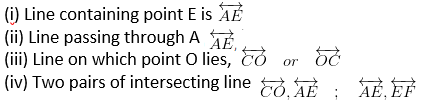# ML Aggarwal Solutions for Class 6 Maths Chapter 10 Basic Geometrical Concept

ML Aggarwal Solutions for Class 6 Maths Chapter 10 Basic Geometrical Concept are available here. When students get doubtful while solving the questions from the textbook, we suggest them to refer to the ML Aggarwal Solutions. Expert personnel at BYJU’S have answered these questions and present a robust and extensive set of solutions in order to assist you with your exam preparation to attain good marks in Maths.

Chapter 10 – Basic Geometrical Concept includes three basic concepts: point, line and plane. In this ML Aggarwal Solutions for Class 6 Maths Chapter 10 students are going to learn solving different types of problems and Basic Geometrical Concept.

## Download the PDF of ML Aggarwal Solutions for Class 6 Maths Chapter 10 Basic Geometrical Concept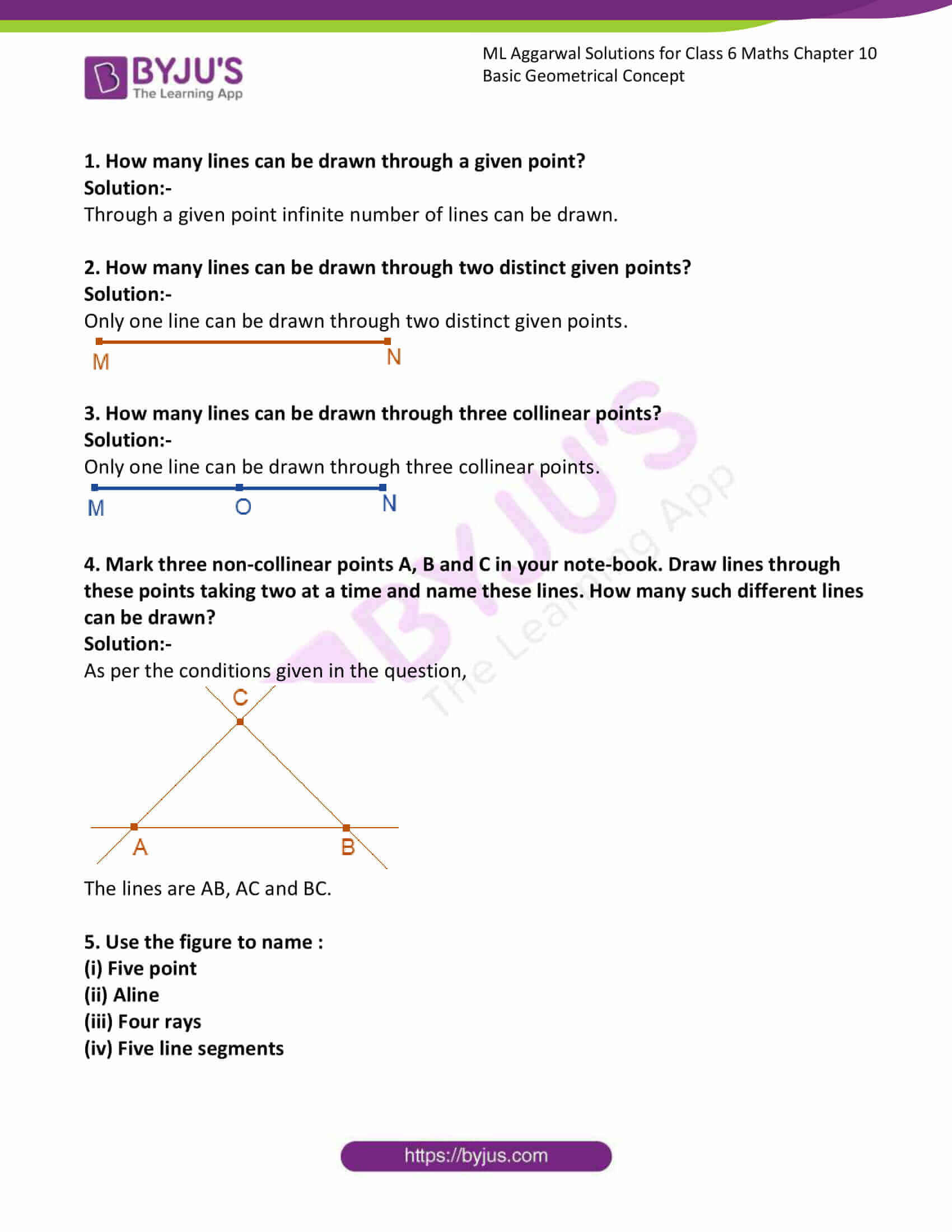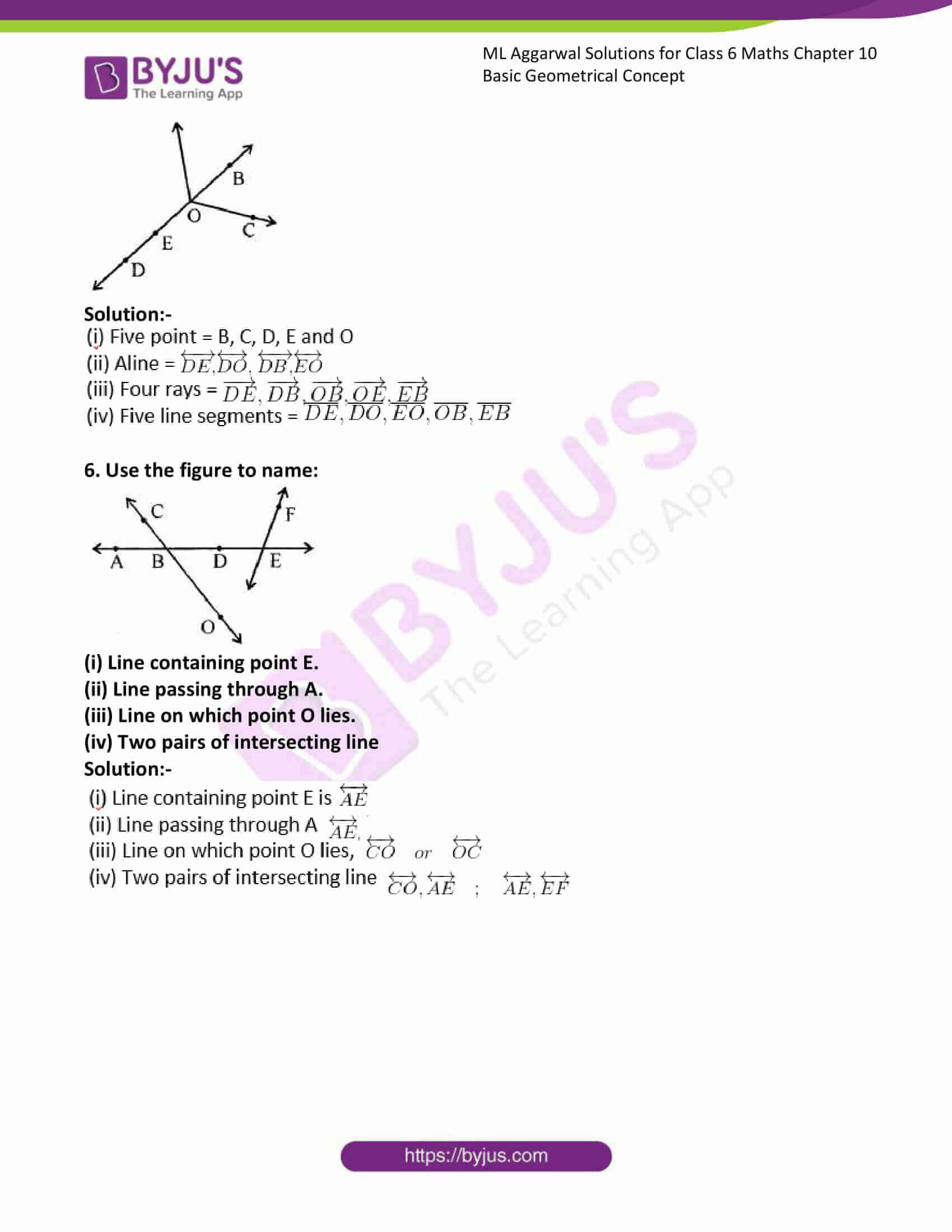### Access answers to ML Aggarwal Solutions for Class 6 Maths Chapter 10 Basic Geometrical Concept

1. How many lines can be drawn through a given point?

Solution:-

Through a given point infinite number of lines can be drawn.

2. How many lines can be drawn through two distinct given points?
Solution:-

Only one line can be drawn through two distinct given points.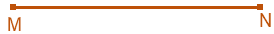3. How many lines can be drawn through three collinear points?
Solution:-

Only one line can be drawn through three collinear points.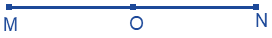4. Mark three non-collinear points A, B and C in your note-book. Draw lines through these points taking two at a time and name these lines. How many such different lines can be drawn?

Solution:-

As per the conditions given in the question,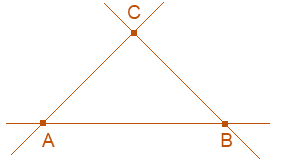The lines are AB, AC and BC.

5. Use the figure to name :
(i) Five point
(ii) Aline
(iii) Four rays
(iv) Five line segments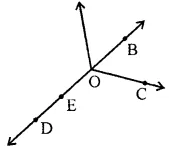Solution:-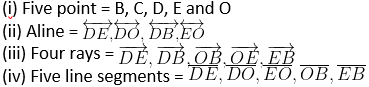6. Use the figure to name: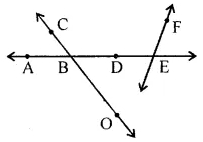(i) Line containing point E.
(ii) Line passing through A.
(iii) Line on which point O lies.
(iv) Two pairs of intersecting line

Solution:-